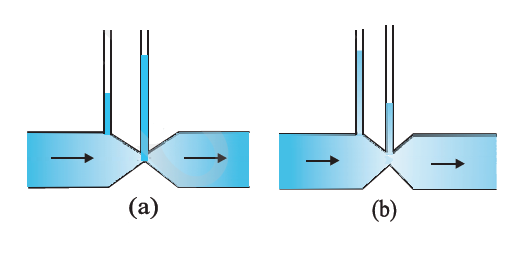## Pages

### Mechanical Properties of Fluids NCERT Solutions Class 11 Physics - Solved Exercise Question 10.15

Question 10.15:
Figures 10.23 (a) and (b) refer to the steady flow of a (non-viscous) liquid. Which of the two figures is incorrect? Why?Solution:
Take the case given in figure (b).Where,
A1 = Area of pipe1
A2 = Area of pipe 2
V1 = Speed of the fluid in pipe1
V2 = Speed of the fluid in pipe 2
From the law of continuity, we have:
A1V1 = A2V2
When the area of cross-section in the middle of the venturimeter is small, the speed of the flow of liquid through this part is more. According to Bernoulli’s principle, if speed is more, then pressure is less.
Pressure is directly proportional to height. Hence, the level of water in pipe 2 is less.
Therefore, figure (a) is not possible.